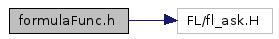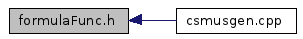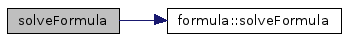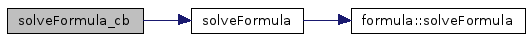# formulaFunc.h File Reference

`#include <FL/fl_ask.H>`

Include dependency graph for formulaFunc.h:This graph shows which files directly or indirectly include this file:## Function Documentation

 void solveFormula ( )

Definition at line 199 of file formulaFunc.h.

References formula::solveFormula().

Referenced by solveFormula_cb().

```00200 {
00201   formula obj;
00202 //  obj.out_formula();
00203   obj.solveFormula();
00204 }
```

Here is the call graph for this function:void solveFormula_cb ( Fl_Widget * , void * )

Definition at line 206 of file formulaFunc.h.

References solveFormula().

Referenced by make_form().

```00207 {
00208     solveFormula();
00209 }
```

Here is the call graph for this function:Generated on Sun Jul 1 20:30:06 2012 for Csmusgen by1.4.7